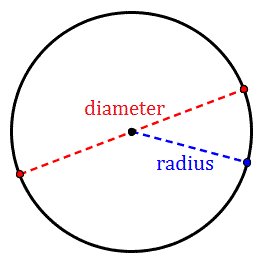Circumference of a Circle

Circumference of a Circle - Definition and Formula

The circumference of a circle is the length of the boundary of the circle. If we cut open the circle and straighten it the length of the boundary will be the measure of the circumference of the circle.A circle is made up of many points arranged equidistant from a single central point and The point is known as the center of the circle.

The distance between the center and any point on the circumference is known as the radius of the circle. The radius is a constant value. It doesn’t change.

The diameter of a circle is the maximum distance between any two points on the circumference. It is twice the radius.

FORMULA FOR CIRCUMFERENCE OF THE CIRCLE

The circumference of a circle is measured in units of length like meter, centimeter, etc. The formula for calculation of circumference is,

Circumference= 2πR

Where R is the radius of the circle,

π (pi) is a constant value.

The value of π = 3.1415926535897…
π or pi is a Greek letter. It is a very important constant in mathematics. It gives the ratio of circumference to the diameter of a circle or the ratio of circumference to twice the radius. For any given circle, this ratio will remain π. It is a constant so whatever be the circumference and the radius the ratio will be equal to π. π is an irrational number hence it cannot be written down as a finite decimal number. Its value is 3.1415926535… Its value goes on to infinite decimal places.

But using an infinite number will make calculations almost impossible. Therefore, for calculations, we can use π =22/7. This makes calculations easier. We can also take the value of π = 3.14. For even more simplification one can take the value of π= 3.
QUESTION: Find the circumference of a circle if the radius is 14 cm. (use π=22/7)

Circumference =2 πR
=2*22/7*14
=88 cm

QUESTIONS:

• 1. If the circumference of a circle is 24 cm, find the radius of this circle.

• 2. Find the circumference of a circle if the radius is 22cm. (use π=22/7)

• 3. Find the circumference of a circle if the radius is 36cm. (use π=3.14)

• 4. If the circumference of a circle is 120cm, find the radius of this circle.

• 5. If the circumference of a circle is 68cm, find the radius of this circle.

• AREA OF A CIRCLE

The area of a circle is the area bounded by the circumference.it is the area inside the circumference. The circumference is the only length. Its width is zero. It is one dimensional.

The area is not one dimensional. The formula for calculation of the area of a circle is given by,
Area= πR2
Where R is the radius of the circle,
π is constant.

Here again, the value of π is non-terminating. Therefore, for easier calculations, we can use π=3.14 or π=22/7.
If we need to calculate the circumference of a circle whose area is given, we will first find the radius from the area and then put it in the formula for circumference.

From the area to get the radius, we should first divide by π and then find the square root of it. This will give us the radius. Next simply, put the value of the radius in the formula for circumference to find the circumference.
One can also find the area of a circle if the circumference is given. For this first, we have to divide the circumference by π and then by 2. This will give us the radius. Then put the radius in the area formula to calculate the area.